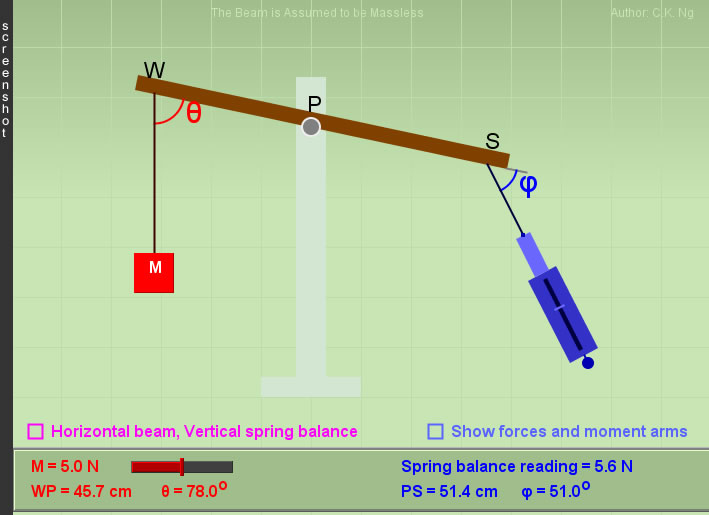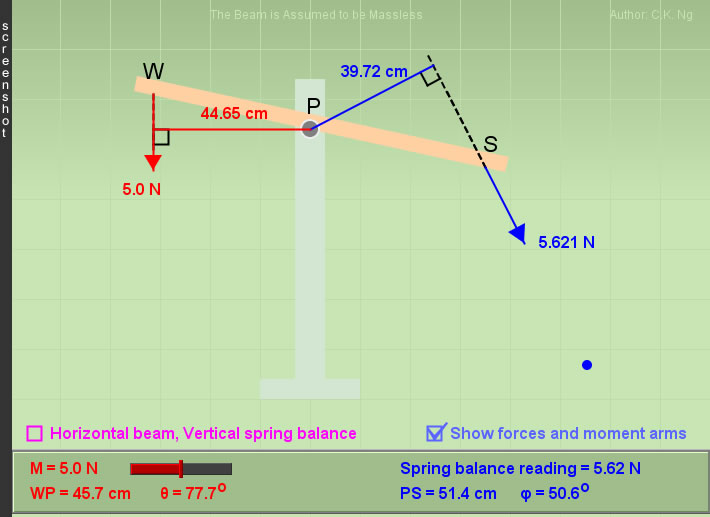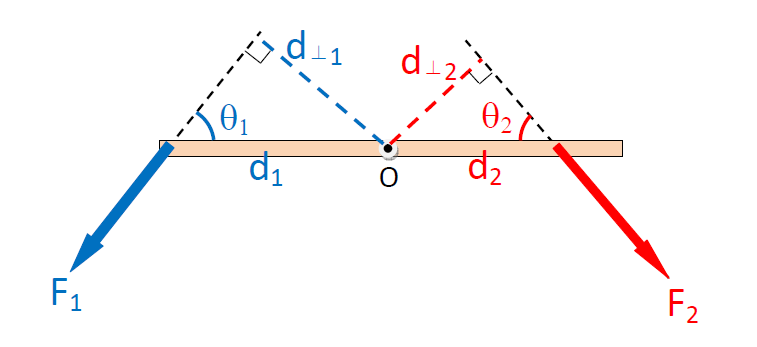Balancing of Turning Moments (Torques)

 The mass of the beam and of the spring balance are neglected. The beam is capable to be turned to any, but not a (nearly) vertical, position. Right-drag to translate the whole system. If unfortunately the beam "collapses", reset the page and start again.

 Internal Links : Balancing a Vertical Pole Sticks falling over
 Definition of Torque : Torque (moment) is defined as τ = F d⊥ = F d sinθ, where d⊥ is called the moment arm of the force F. That will produce a clockwise (anticlockwise) rotation about a pivot is called a clockwise (anticlockwise) torque about that pivot.In the above diagram, ↻↻↻  clockwise torque about O = F2 d⊥2  ↻↻↻ ↺↺↺  anticlockwise torque about O = F1 d⊥1  ↺↺↺ Conditions for Static Equilibrium Suppose all the forces are on the same plane. The vector sum of the forces is zero. The sum of the clockwise torques about any point (an assumed pivot) must be equal to the sum of the anticlockwise torques about that point.

 The author (Chiu-king Ng) has the copyright on all the simulations in this website. Email phyAA@phy.hk, where AA is the prime number following 7. Last Update:2019-9-15